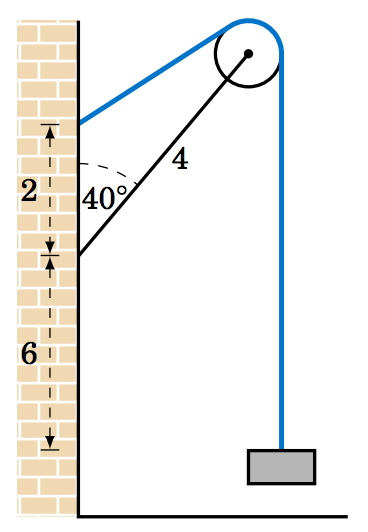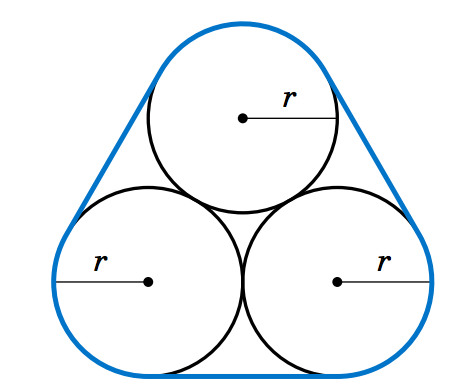$$\newcommand{\id}{\mathrm{id}}$$ $$\newcommand{\Span}{\mathrm{span}}$$ $$\newcommand{\kernel}{\mathrm{null}\,}$$ $$\newcommand{\range}{\mathrm{range}\,}$$ $$\newcommand{\RealPart}{\mathrm{Re}}$$ $$\newcommand{\ImaginaryPart}{\mathrm{Im}}$$ $$\newcommand{\Argument}{\mathrm{Arg}}$$ $$\newcommand{\norm}{\| #1 \|}$$ $$\newcommand{\inner}{\langle #1, #2 \rangle}$$ $$\newcommand{\Span}{\mathrm{span}}$$

$$\newcommand{\vecs}{\overset { \rightharpoonup} {\mathbf{#1}} }$$ $$\newcommand{\vecd}{\overset{-\!-\!\rightharpoonup}{\vphantom{a}\smash {#1}}}$$$$\newcommand{\id}{\mathrm{id}}$$ $$\newcommand{\Span}{\mathrm{span}}$$ $$\newcommand{\kernel}{\mathrm{null}\,}$$ $$\newcommand{\range}{\mathrm{range}\,}$$ $$\newcommand{\RealPart}{\mathrm{Re}}$$ $$\newcommand{\ImaginaryPart}{\mathrm{Im}}$$ $$\newcommand{\Argument}{\mathrm{Arg}}$$ $$\newcommand{\norm}{\| #1 \|}$$ $$\newcommand{\inner}{\langle #1, #2 \rangle}$$ $$\newcommand{\Span}{\mathrm{span}}$$ $$\newcommand{\id}{\mathrm{id}}$$ $$\newcommand{\Span}{\mathrm{span}}$$ $$\newcommand{\kernel}{\mathrm{null}\,}$$ $$\newcommand{\range}{\mathrm{range}\,}$$ $$\newcommand{\RealPart}{\mathrm{Re}}$$ $$\newcommand{\ImaginaryPart}{\mathrm{Im}}$$ $$\newcommand{\Argument}{\mathrm{Arg}}$$ $$\newcommand{\norm}{\| #1 \|}$$ $$\newcommand{\inner}{\langle #1, #2 \rangle}$$ $$\newcommand{\Span}{\mathrm{span}}$$

These are homework exercises to accompany Corral's "Elementary Trigonometry" Textmap. This is a text on elementary trigonometry, designed for students who have completed courses in high-school algebra and geometry. Though designed for college students, it could also be used in high schools. The traditional topics are covered, but a more geometrical approach is taken than usual. Also, some numerical methods (e.g. the secant method for solving trigonometric equations) are discussed.

## 4.1 Exercise

For Exercises 1-5, convert the given angle to radians.

4.1.1 $$4^\circ$$

4.1.2 $$15^\circ$$

4.1.3 $$130^\circ$$

4.1.4 $$275^\circ$$

4.1.5 $$-108^\circ$$

For Exercises 6-10, convert the given angle to degrees.

4.1.6 $$4$$ rad

4.1.7 $$\dfrac{\pi}{5}$$ rad

4.1.8 $$\dfrac{11\pi}{9}$$ rad

4.1.9 $$\dfrac{29\pi}{30}$$ rad

4.1.10 $$35$$ rad

4.1.11 Put your calculator in radian mode and take the cosine of $$0$$. Whatever the answer is, take its cosine. Then take the cosine of the new answer. Keep repeating this. On most calculators after about $$50$$-$$60$$ iterations you should start to see the same answer repeating. What is that number? Try starting with a number different from $$0$$. Do you get the same answer repeating after roughly the same number of iterations as before? Try the same procedure in degree mode, starting with $$0^\circ$$. Does the same thing happen? If so, does it take fewer iterations for the answer to start repeating than in radian mode, or more?

## 4.2 Exercise

For Exercises 1-4, find the length of the arc cut off by the given central angle $$\theta$$ in a circle of radius $$r$$.

4.2.1 $$\theta=0.8$$ rad, $$r=12$$ cm

4.2.2 $$\theta=171^\circ$$, $$r=8$$ m

4.2.3 $$\theta=\pi$$ rad, $$r=11$$ in

4.2.4 A central angle in a circle of radius $$2$$ cm cuts off an arc of length $$4.6$$ cm. What is the measure of the angle in radians? What is the measure of the angle in degrees?

4.2.5 The centers of two belt pulleys, with radii of $$3$$ inches and $$6$$ inches, respectively, are $$13$$ inches apart. Find the total length $$L$$ of the belt around the pulleys.

4.2.6 In Figure 4.2.5 one end of a $$4$$ ft iron rod is attached to the center of a pulley with radius $$0.5$$ ft. The other end is attached at a $$40^\circ$$ angle to a wall, at a spot $$6$$ ft above the lower end of a steel wire supporting a box. The other end of the wire comes out of the wall straight across from the top of the pulley. Find the length $$L$$ of the wire from the wall to the box.Figure 4.2.5 Exercise 6Figure 4.2.6 Exercise 7

4.2.7 Figure 4.2.6 shows the same setup as in Exercise 6 but now the wire comes out of the wall $$2$$ ft above where the rod is attached. Find the length $$L$$ of the wire from the wall to the box.

4.2.8 Find the total length $$L$$ of the figure eight shape in Figure 4.2.7.Figure 4.2.7

4.2.9 Repeat Exercise 8 but with the circle at $$A$$ having a radius of $$3$$ instead of $$2$$. (Hint: Draw a circle of radius $$5$$ centered at $$A$$, then draw a tangent line to that circle from $$B$$.)

4.2.10 Suppose that in Figure 4.2.7 the lines do not criss-cross but instead go straight across, as in a belt pulley system. Find the total length $$L$$ of the resulting shape.

4.2.11 Find the lengths of the two arcs cut off by a chord of length $$3$$ in a circle of radius $$2$$.

4.2.12 Find the perimeter of a regular dodecagon (i.e. a $$12$$-sided polygon with sides of equal length) inscribed inside a circle of radius $$\frac{1}{2}$$. Compare it to the circumference of the circle.

## 4.3 Exercise

For Exercises 1-3, find the area of the sector for the given angle $$\theta$$ and radius $$r$$.

4.3.1 $$\theta = 2.1$$ rad, $$r = 1.2$$ cm

4.3.2 $$\theta = \frac{3\pi}{7}$$ rad, $$r = 3.5$$ ft

4.3.3 $$\theta = 78^\circ$$, $$r = 6$$ m

4.3.4 The centers of two belt pulleys, with radii of $$3$$ cm and $$6$$ cm, respectively, are $$13$$ cm apart. Find the total area $$K$$ enclosed by the belt.

4.3.5 In Exercise 4 suppose that both belt pulleys have the same radius of $$6$$ cm. Find the total area $$K$$ enclosed by the belt.

4.3.6 Find the area enclosed by the figure eight in Exercise 8 from Section 4.2.

For Exercises 7-9, find the area of the sector for the given radius $$r$$ and arc length $$s$$.

4.3.7 $$r = 5$$ cm, $$s = 2$$ cm

4.3.8 $$r = a$$, $$s = a$$

4.3.9 $$r = 1$$ cm, $$s = \pi$$ cm

For Exercises 10-12, find the area of the segment formed by a chord of length $$a$$ in a circle of radius $$r$$.

4.3.10 $$a = 4$$ cm, $$r = 4$$ cm

4.3.11 $$a = 1$$ cm, $$r = 5$$ cm

4.3.12 $$a = 2$$ cm, $$r = 5$$ cm

4.3.13 Find the area of the shaded region in Figure 4.3.7.Figure 4.3.7 Exercise 13

4.3.14 Find the area of the shaded region in Figure 4.3.8. (Hint: Draw two central angles.)Figure 4.3.8 Exercise 14

4.3.15 Find the area of the shaded region in Figure 4.3.9.Figure 4.3.9 Exercise 15

4.3.16 The centers of two circles are $$4$$ cm apart, with one circle having a radius of $$3$$ cm and the other a radius of $$2$$ cm. Find the area of their intersection.

4.3.17 Three circles with radii of $$4$$ m, $$2$$ m, and $$1$$ m are externally tangent to each other. Find the area of the curved region between the circles, as in Figure 4.3.10. (Hint: Connect the centers of the circles.)Figure 4.3.10 Exercise 17Figure 4.3.11 Exercise 18

4.3.18 Show that the total area enclosed by the loop around the three circles of radius $$r$$ in Figure 4.3.11 is $$\;(\pi + 6 + \sqrt{3})\,r^2$$.

4.3.19 For a fixed central angle $$\theta$$, how much does the area of its sector increase when the radius of the circle is doubled? How much does the length of its intercepted arc increase?

## 4.4 Exercise

For Exercises 1-6, assume that a particle moves along a circle of radius $$r$$ for a period of time $$t$$. Given either the arc length $$s$$ or the central angle $$\theta$$ swept out by the particle, find the linear and angular speed of the particle.

4.4.1 $$r=4$$ m, $$t=2$$ sec, $$\theta=3$$ rad

4.4.2 $$r=8$$ m, $$t=2$$ sec, $$\theta=3$$ rad

4.4.3 $$r=7$$ m, $$t=3.2$$ sec, $$\theta=172^\circ$$

4.4.4 $$r=1$$ m, $$t=1.6$$ sec, $$s=3$$ m

4.4.5 $$r=2$$ m, $$t=1.6$$ sec, $$s=6$$ m

4.4.6 $$r=1.5$$ ft, $$t=0.3$$ sec, $$s=4$$ in

4.4.7 An object moves at a constant linear speed of $$6$$ m/sec around a circle of radius $$3.2$$ m. How large of a central angle does it sweep out in $$1.8$$ seconds?

4.4.8 Two interlocking gears have outer radii of $$6$$ cm and $$9$$ cm, respectively. If the smaller gear rotates at $$40$$ rpm, how fast does the larger gear rotate?

4.4.9 Three interlocking gears have outer radii of $$2$$ cm, $$3$$ cm, and $$4$$ cm, respectively. If the largest gear rotates at $$16$$ rpm, how fast do the other gears rotate?

4.4.10 In Example 4.17, does equation 4.11 still hold if the radii $$r_1$$ and $$r_2$$ are replaced by the number of teeth $$N_1$$ and $$N_2$$, respectively, of the two gears as shown in Figure 4.4.2?

4.4.11 A $$78$$ rpm music record has a diameter of $$10$$ inches. What is the linear speed of a speck of dust on the outer edge of the record in inches per second?

4.4.12 The centripetal acceleration $$\alpha$$ of an object moving along a circle of radius $$r$$ with a linear speed $$\nu$$ is defined as $$\;\alpha = \frac{\nu^2}{r}$$. Show that $$\;\alpha = \omega^2 \,r$$, where $$\omega$$ is the angular speed.